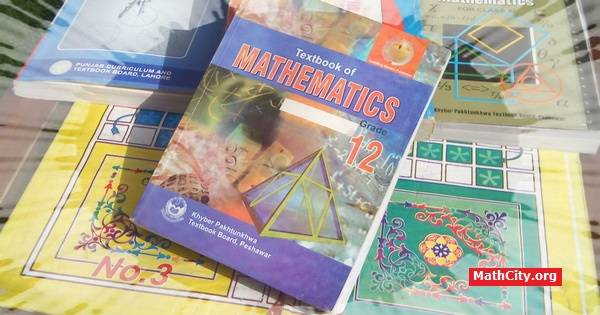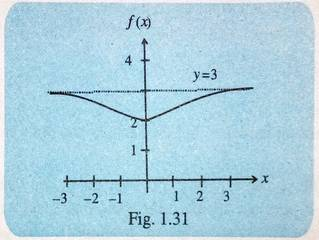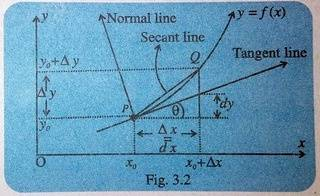# FSc Part 2 (KPK Boards)Notes of FSc Part 2 of “A Textbook of Mathematics For Class XII” published by Khyber Pakhtunkhwa Textbook Board, Peshawar. We are posting the notes chapter-wise. These notes are shared as open educational resources. This page will be continuously updated.#### Objectives

After reading this unit the students will be able to:

• identify the domain and range of a functions through graphs.
• draw the graph of modulus function and identify its domain and range.
• recognize the composition of a function and then to find out the composition of two functions.
• describe the inverse of a function and then to find out the inverse of composition of two functions.
• recognize the algebraic and transcendental functions as well as the concepts of explicit, implicit and parametric functions.
• display graphically the explicit, implicit and parametric functions as well as the compound functions.
• introduce the limit of a function with respect to real number intervals on the real number line, the open and closed intervals and its location on a real number line.
• explain the meaning of x tends to zero, x tends to a and x tends to infinity
• define the limit of a sequence when the limit of a sequence with nth term is given.
• define the limit of a function and the statement of theorems on limits of sum, difference, product and quotient of functions.
• evaluate the limits of a function in case of some special functions.
• evaluate the limit of algebraic, exponential and trigonometric functions.
• introduce the continuous and discontinuous functions.
• recognize the left hand and right hand limits through examples.
• define the continuity of a function at a point and in an interval.
• test the continuity and discontinuity of a function a point and in an interval.

#### Objectives

After reading this unit the students will be able to:

• distinguish between independent and dependent variables.
• estimate the change in the dependent variable, when the independent variable is incremented or decremented.
• explain the concepts of a rate of change.
• define the derivative of a function as an instantaneous rate of change of variable with respect to another variable.
• define derivative or differential coefficient of a function.
• differentiate $y=x^n$ and $y=(ax+b)^n$ by first principle rule.
• introduce the theorems of differentiation, such as the derivative of a constant function, the derivative of any constant multiple of a function, the derivative of a sum or difference of two functions, the derivative of the product of two functions and the derivative of a quotient of two functions.
• apply theorems of differentiation in solving problems.
• introduce chain rule of differentiation in different situations.
• introduce implicit differentiation of a function.
• introduce differentiation of trigonometric and inverse trigonometric functions.
• introduce differentiation of exponential and logarithmic functions.
• introduce the differentiation of hyperbolic and inverse hyperbolic functions.#### Objectives

After reading this unit the students will be able to:

• Integration

#### Objectives

After reading this unit the students will be able to:

• Differentiation
ch04-differentiation-of-vector-functions-fsc2-kpk.pdf |

#### Objectives

After reading this unit the students will be able to:

• Integration
ch05-integration-fsc2-kpk.pdf |

#### Objectives

This unit tells us, how to:

• define the differential equation, its order, degree, general and particular solutions, and its identification as linear and nonlinear ordinary differential equations.
• demonstrate the concept in forming a differential equation.
• solve the first order linear and nonlinear ordinary differential equations by separable variable form, and homogenuous form and then how to reduce differential equations in standard form of homogenuous.
• solve the real life problems related to differential equations.
• define the orthogonal trajectories and then how to show the orthogonal trajectories of the two families of curve.
ch09-differential-equations-fsc2-kpk.pdf |

• fsc/kpk_fsc_part_2